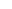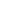# Numpy Floor, Explained

In this tutorial, I’ll explain how to use the Numpy floor function, which is sometime called np.floor.

I’ll explain the syntax of np.floor and how the function works.

I’ll also show you clear, step by step examples of how to use it.

If you need something specific, you can click on any of the following links, and it will take you to the appropriate section in the tutorial.

Having said that, it’s probably best if you read the whole tutorial. Everything will probably make more sense that way.

## A Quick Introduction to Numpy Floor

First of all, let’s just start off with an overview of the Numpy Floor function.

The Numpy Floor function is a function for the Numpy package.

Numpy is a Python package that enables you to work with numeric data that’s arranged in an array format.

Numpy arrays contain numeric data, arranged in a grid-like format. As an example, a 2D array looks something like this:Remember though, Numpy arrays can have 1-dimension, 2-dimensions, or n-dimensions.

Numpy has a variety of functions to create arrays with various properties, including the Numpy array function, the Numpy zeros function, Numpy ones, Numpy arange, and more.

### Numpy Floor is a Math Function for Numpy

So what then is the Numpy Floor function?

In addition to having functions for creating Numpy arrays, the Numpy package also has a variety of functions for operating on Numpy arrays.

Specifically, Numpy has several functions for performing numerical operations on Numpy arrays (remember: Numpy arrays are filled with numerical data).

The np.floor function is one of these functions.

### Numpy Floor Computes the “Floor” of Floating Point Numbers

A little more specifically, the Numpy floor function computes the floor of the individual numbers in a Numpy array.

Remember, the floor function takes a real numberas an input and returns the largest integer that is less than or equal to.

In mathematical notation, this function is typically denoted as.

In Numpy, we can use the floor function to compute the “floor” values of floating point numbers (i.e., real numbers).So for example, we can compute the floor of 2.17 as:But not only can Numpy floor compute the floor of single values, it can also operate on full Numpy arrays.

#### Numpy Floor Computes Floor Values Element-Wise on Numpy Arrays

When you use Numpy floor on a Numpy array, the np.floor function computes the floors of every value in the array, element wise.

So if you have a 1-dimensional Numpy array, np.floor will operate on all of the values:For the most part, the np.floor function is simple. It computes the floor values of single numbers, or the numbers contained in a Numpy array.

## The syntax of np.floor

Now that you’ve learned what the floor function does, let’s take a look at the syntax.

#### A quick note

One quick note before we look at the syntax.

Whenever we use a Python package, we need to import that package.

And how we import the package will import exactly how we type the syntax.

In this case, with Numpy, the common convention is to import Numpy with the alias ‘`np`‘. So we commonly import Numpy with this code:

```import numpy as np
```

When we import Numpy this way, that will enable us to call Numpy functions with the prefix ‘`np.`‘ before the name of the function.

As I mentioned, this is the common convention among Python programmers when using Numpy, and all of our syntax will assume this import convention.

### np.floor Syntax

The syntax for np.floor is very simple.

We call the function as `np.floor()`.

Then, inside the parenthesis, we can provide either a single number or a Numpy array of many numbers.Having said that, let’s take a closer look at the input parameter for np.floor.

### The Parameters of np.floor

There’s really only one commonly used parameter for the np.floor function, that’s the input parameter. This input parameter is sometimes denoted as `x`.

Keep in mind that np.floor does have some other parameters, including `out` and `where`. However, these other parameters are very rarely used, so we’re not going to cover them in this tutorial.

###### `x` (required)

Let’s talk a little more specifically about the `x` parameter.

The `x` parameter enables you to specify an input to the np.floor function.

The input (i.e., the argument to the `x` parameter) can be a single number.

It can also be a Numpy array or an “array like” object. Array like objects include things like Python lists and tuples.

So as an input to the np.floor function, you can provide a number, a Numpy array, or an array like object. They’ll all work (you’ll see some examples in the examples section).

### The Output of np.floor

If the input to np.floor is a Numpy array or array-like object, then the output of Numpy floor is a new Numpy array. This new output array will contain the floor value of each individual element of the input array.

If the input to np.floor is a single value, then the output will be a single value (i.e., the floor of the input).

## Examples of How to Use Numpy Floor

Now that you’ve looked at the syntax, let’s take a look at some examples.

Examples:

If you click on any of the links above, it will take you to the appropriate example.

#### Run this code first

One quick thing before we get started with the examples.

As explained in the section about syntax, how exactly we call the function depends on how we’ve imported Numpy. The common convention among Python programmers is to import Numpy with the alias ‘`np`‘.

That said, before you run the following examples, you need to import Numpy with this code:

```import numpy as np
```

Once you’ve done that, you’ll be ready to get started.

### EXAMPLE 1: Compute floor of a single value

First, let’s just compute the floor of a single value.

Here, we’ll compute the floor of the number 2.17.

```np.floor(2.17)
```

OUT:

```2.
```
###### Explanation

This example is very simple.

Here, we’ve used the value 2.17 as the input to the function. This is a floating point number (a “real” number, AKA a decimal number).

As the output, Numpy floor has computed the smallest integer value that’s less than or equal to the input. In this case, the value is 2.

(One small note: even though the output number is an integer, the Python data type of the output is `float`!)

### EXAMPLE 2: Compute floor of values in a Python list

Next, let’s compute the floors of the values in a Python list.

```np.floor([1.1, 2.2, 3.3, 4.4])
```

OUT:

```array([1., 2., 3., 4.])
```
###### Explanation

This is very simple.

The input to the function is the Python list `[1.1, 2.2, 3.3, 4.4]`. Each of these values in the input array is a floating point number with a decimal value.

When np.float operates on this list, it computes the floor of each value, element wise.

It returns the output as a Numpy array that contains those computed floor values.

(But be careful: the data type of values in the output array is actually `float`.)

### EXAMPLE 3: Compute the floors of values in a Numpy array

Let’s do one last example.

Here, we’ll compute the floors of the values of Numpy array.

First, we need to create the Numpy array.

```array_1d = np.array([1.1, 2.2, 3.3, 4.4, 5.5])
```

And we can print the values:

```print(array_1d)
```

OUT:

```[1.1 2.2 3.3 4.4 5.5]
```

Next, we’ll compute the floors of each of these values:

```np.floor(array_1d)
```

OUT:

```array([1., 2., 3., 4., 5.])
```
###### Explanation

Again, this is fairly simple and it’s very similar to example 2.

The input is a Numpy array. The input array has several floating point numbers.

When we pass this array to np.floor, the function produces a new Numpy array that contains the floors of the values of the input array. The np.floor function computes the floor of each value of the input, element wise, and puts those values in the new output array.

Keep in mind that here, we’ve used a 1-dimensional array. However, we could also provide a 2-dimensional array or an n-dimensional array as an input. The np floor function will work the same way … it will compute the floors of the input values in an element wise fashion, and put those floor values in a new output array of the same shape.

Do you have other questions about the Numpy floor function?

If so, just leave your question in the comments section at the bottom of the page.

The examples here about Numpy floor are pretty simple and easy to understand.

But other parts of Numpy can be a lot more complicated.

If you’re serious about learning Numpy (and serious about data science in Python), you should consider joining our premium course called Numpy Mastery.

Numpy Mastery will teach you everything you need to know about Numpy, including:

• How to create Numpy arrays
• How to use the Numpy random functions
• What the “Numpy random seed” function does
• How to reshape, split, and combine your Numpy arrays
• How to perform mathematical operations on Numpy arrays
• and more …

Moreover, it will help you completely master the syntax within a few weeks. We’ll show you a practice system that will enable you to memorize all of the Numpy syntax you learn. If you have trouble remembering Numpy syntax, this is the course you’ve been looking for.

Find out more here:

Joshua Ebner

Joshua Ebner is the founder, CEO, and Chief Data Scientist of Sharp Sight. Prior to founding the company, Josh worked as a Data Scientist at Apple. He has a degree in Physics from Cornell University.